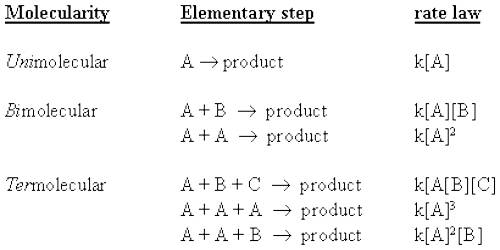# Molecularity with Bimolecular Step

Molecularity with Bimolecular Step

The molecularity is the number of particles (molecules, atoms or ions) on the reactant side of an elementary reaction. We do not talk of molecularity of a net or overall reaction. Molecularity is not related to the stoichiometric equation of a reaction.

For an elementary step of the reaction the rate is proportional to the concentration of each reactant species in the step. Hence the molecularity of each elementary step of the reaction is the same as the order of that step. A bimolecular step will have second-order kinetics. This, however, does not give the overall order of the reaction.

Let consider the reaction –

2NO (g) + 2 H2 (g) → 2 H2O (g) + N2 (g)

Experimentally it is found to be a third-order reaction having the rate law.

then, rate = k [NO]2 [H2]

It appears that the reaction proceeds by the three-step mechanism

2NO (g) → N2O2 (g)

N2O2 (g) + H2 (g) → N2O (g) + H2O (g)

N2O (g) + H2 (g) → N2 (g) + H2O (g)

How the above mechanism came to be proposed can be illustrated by taking a general reaction of the above type, e.g.,

2A + B → C + D

Let us say that the experimental rate law for this reaction is-

rate = k [A]2 [B]

If we assume that the reaction proceeds by a one step mechanism in which two molecules of A and one of B collide together simultaneously, i.e., a termolecuular collision takes place, and then the rate equation would be as shown. However, termolecular collisions being rare one would look for an alternative mechanism which is more realistic. Bimolecular collisions being more common it would be more plausible to see if use of bimolecular elementary steps lead to the experimental rate law.

One possible set of elementary steps would be-

(1) 2A → S2

(2) A2 +B → C + D

In this mechanism two molecules of A first combine to form a reaction intermediate A2 which then reacts with B to form the products C and D. We can see that the sum of the two steps gives the overall reaction. Both of these reactions are unlikely to proceed at the same rate. If it is assumed that step (1) is slow, and once the intermediate is formed it reacts immediately with B to give the products. Elementary reaction (1) is then the rate- determining step (in this case the bimolecular collision between two A Molecules) and we can predict that the rate law for this elementary step would be –

rate = k [A]2